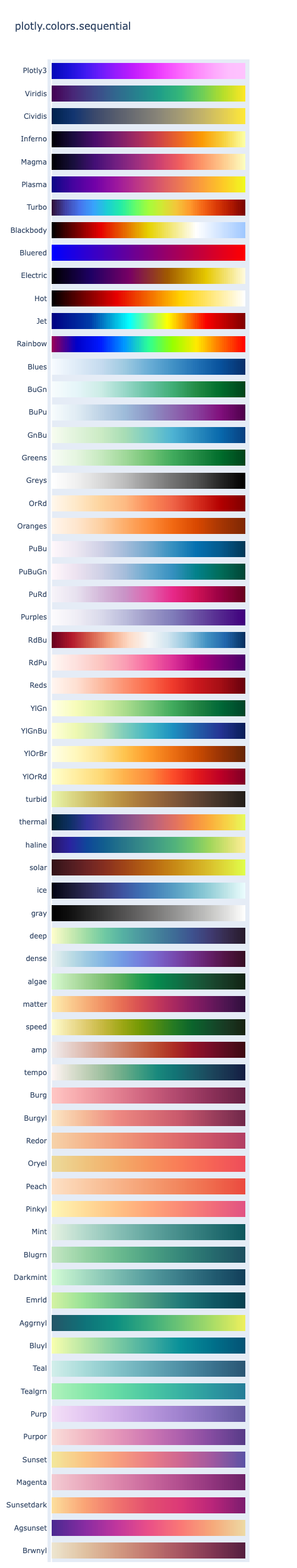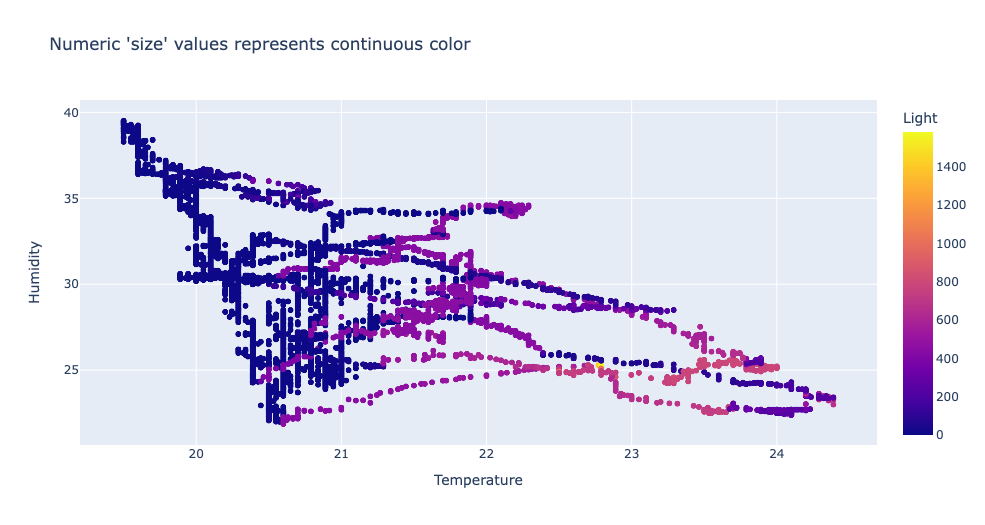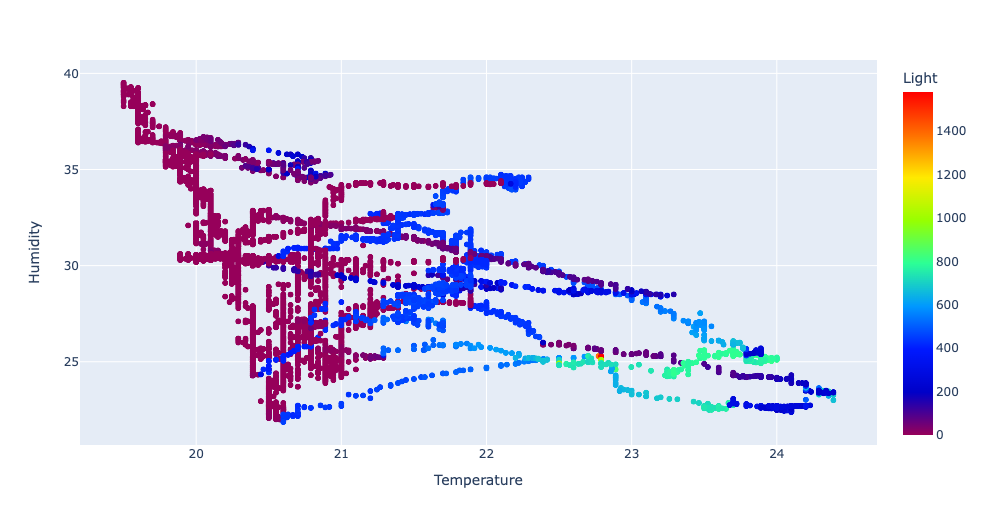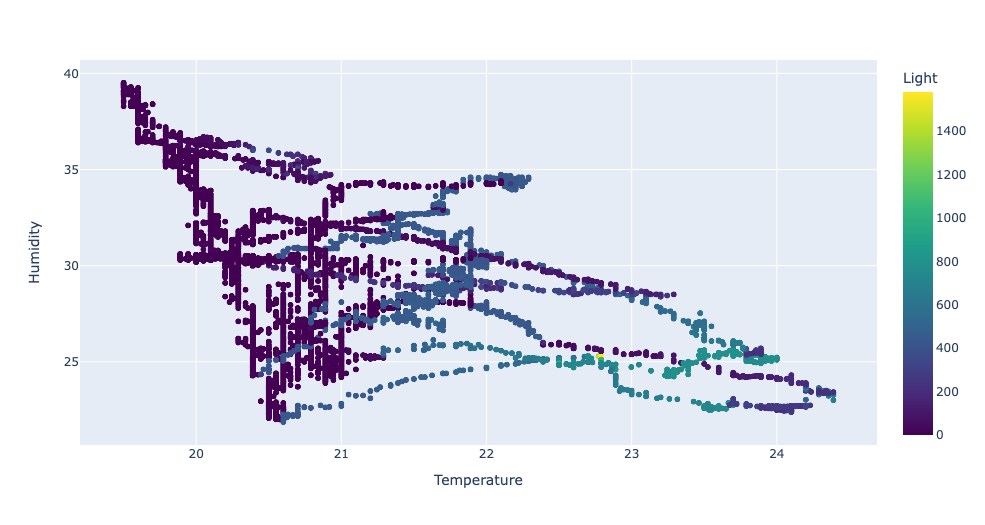Open in App
Not now

# Built-in Continuous Color Scales in Python Plotly

• Last Updated : 28 Feb, 2022

Plotly has built-in discrete and continuous color scales. This article is about discrete color scales. A color continuous scale input is accepted by several Plotly Express functions, and many trace types have a color scale property in their schema. Plotly has a wide variety of built-in continuous color scales that can be referenced in Python code when specifying the arguments, either by name or by reference.

Code to print out the names of the color scales:

## Python3

 `#import packages ` `import` `plotly.express as px ` ` `  `plotly_colorscales ``=` `px.colors.named_colorscales() ` ` `  `# printing color scales ` `print``(plotly_colorscales) `

Output:

[‘aggrnyl’, ‘agsunset’, ‘blackbody’, ‘bluered’, ‘blues’, ‘blugrn’, ‘bluyl’, ‘brwnyl’, ‘bugn’, ‘bupu’,’burg’, ‘burgyl’, ‘cividis’, ‘darkmint’, ‘electric’, ’emrld’, ‘gnbu’, ‘greens’, ‘greys’, ‘hot’, ‘inferno’,’jet’, ‘magenta’, ‘magma’, ‘mint’, ‘orrd’, ‘oranges’, ‘oryel’, ‘peach’, ‘pinkyl’, ‘plasma’, ‘plotly3′,’pubu’, ‘pubugn’, ‘purd’, ‘purp’, ‘purples’, ‘purpor’, ‘rainbow’, ‘rdbu’, ‘rdpu’, ‘redor’, ‘reds’,’sunset’, ‘sunsetdark’, ‘teal’, ‘tealgrn’, ‘turbo’, ‘viridis’, ‘ylgn’, ‘ylgnbu’, ‘ylorbr’, ‘ylorrd’,’algae’, ‘amp’, ‘deep’, ‘dense’, ‘gray’, ‘haline’, ‘ice’, ‘matter’, ‘solar’, ‘speed’, ‘tempo’, ‘thermal’,’turbid’, ‘armyrose’, ‘brbg’, ‘earth’, ‘fall’, ‘geyser’, ‘prgn’, ‘piyg’, ‘picnic’, ‘portland’, ‘puor’,’rdgy’, ‘rdylbu’, ‘rdylgn’, ‘spectral’, ‘tealrose’, ‘temps’, ‘tropic’, ‘balance’, ‘curl’, ‘delta’, ‘oxy’, ‘edge’, ‘hsv’, ‘icefire’, ‘phase’, ‘twilight’, ‘mrybm’, ‘mygbm’]

Code to view the built-in sequential color scales in plotly.colors.sequential module. Swatches_sequential() method is used to view the color scales. The method returns a plot of all sequential color scales:

## Python3

 `# importing packages ` `import` `plotly.express as px ` ` `  `fig ``=` `px.colors.sequential.swatches_continuous() ` `fig.show() `

Output:### Example 1:

A scatter plot is plotted where the color of the scatterplot depends on the column ‘Light’. ‘size’ values represent continuous color.

## Python3

 `# import packages and libraries ` `import` `pandas as pd ` `from` `matplotlib ``import` `pyplot as plt ` `import` `numpy as np ` `import` `plotly.express as px ` ` `  `# reading the dataset ` `df ``=` `pd.read_csv(``'weather.csv'``, encoding``=``'UTF-8'``) ` ` `  `# plot a scatterplot ` `fig ``=` `px.scatter(df, x``=``"Temperature"``, y``=``'Humidity'``, color``=``'Light'``, ` `                 ``title``=``"Numeric 'size' values represents continuous color"``) ` ` `  ` `  `fig.show() `

Output:### Example 2:

The same example is repeated again but in the px. scatter() method we include an extra parameter ‘color_continous_scale’, name of the colour scale is given as input. px.colors.sequential contain color scales. In the given example Rainbow is the name of the color scale.

## Python3

 `# import packages and libraries ` `import` `pandas as pd ` `from` `matplotlib ``import` `pyplot as plt ` `import` `numpy as np ` `import` `plotly.express as px ` ` `  `# reading the dataset ` `df ``=` `pd.read_csv(``'weather.csv'``, encoding``=``'UTF-8'``) ` ` `  `# creating a scatterplot ` `fig ``=` `px.scatter(df, x``=``"Temperature"``, y``=``'Humidity'``, color``=``'Light'``, ` `                 ``color_continuous_scale``=``px.colors.sequential.Rainbow) ` ` `  ` `  `fig.show() `

Output:### Example 3:

We can also specify the name of the color scale by using the name of the continuous color scale as a string. ‘Viridis’ is the name of the color scale.

## Python3

 `# import packages and libraries ` `import` `pandas as pd ` `from` `matplotlib ``import` `pyplot as plt ` `import` `numpy as np ` `import` `plotly.express as px ` ` `  `# reading the dataset ` `df ``=` `pd.read_csv(``'weather.csv'``, encoding``=``'UTF-8'``) ` ` `  `# creating a scatterplot ` `fig ``=` `px.scatter(df, x``=``"Temperature"``, y``=``'Humidity'``, color``=``'Light'``, ` `                 ``color_continuous_scale``=``'Viridis'``) ` ` `  ` `  `fig.show() `

Output:My Personal Notes arrow_drop_up
Related Articles